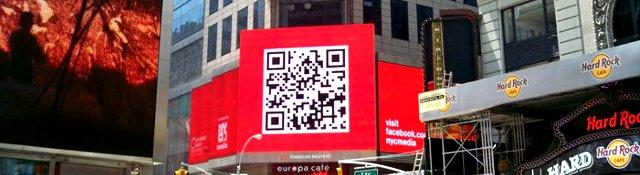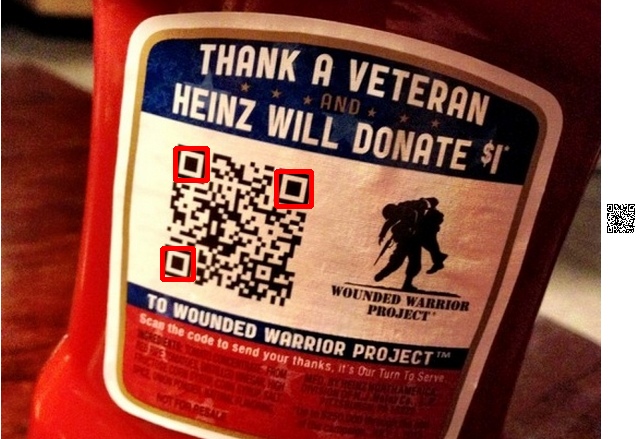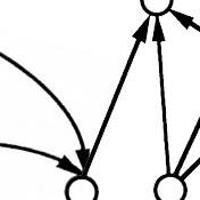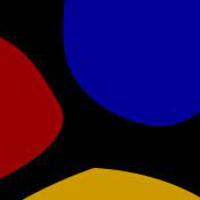## Scanning QR Codes

Recognize QR Codes in images from scratch. We'll do all the bit math to figure out the location markers and then read data from the black/white array.

## Extracting bits from the QR code

Series: Scanning QR Codes:

1. Introduction
2. Locating the QR code
3. Verifying the finder patterns
4. Extracting bits from the QR code

Until now, we've been able to get the finder patterns and get the centers of the three. Now, let's take a look at actually deciphering the contents of the QR code. The actual decoding process is a bit involved and requires Solomon-Reed codes. For the purpose of this tutorial, we'll read the pixels and translate them into bits.

We'll be adding a bunch of new functions to our QR code reader class to help us extract just the code out.

```class qrReader {
public:
...
bool findAlignmentMarker(const Mat& img);
void getTransformedMarker(const Mat &img, Mat& output);
void extractBits(const Mat &img);

private:
bool checkRatio(int* stateCount);
...
int computeDimension(Point2f tl, Point2f tr, Point2f bl, float moduleSize);
...

vector<float> estimatedModuleSize;
int dimension = -1;
}
```

### Computing the dimensions of a code

Any valid QR Code is always a square with 21x21, 25x25, 29x29, etc modules along each dimension. These are called "versions" of a QR code. Version 1 corresponds to 21x21. Version 2 corresponds to 25x25, etc. The dimensions always have a remainder of 1 when divided by four.

Thus, we should be able to compute the dimensions of the code using the finder pattern locations we've computed until now.

```int qrReader::computeDimension(Point2f tl, Point2f tr, Point2f bl, float moduleSize) {
// The dimension is always a square of dimension 21*21, 25*25, 29*29, etc

Point2f diff_top = tl-tr;
Point2f diff_left = tl-bl;

// Calculate the distance between the top-* and *-left points
float dist_top = sqrt(diff_top.dot(diff_top));
float dist_left = sqrt(diff_left.dot(diff_left));
```

Once we have the approximate number of pixels in each dimension, we can convert that into the number of modules:

```    int width = (int)round(dist_top/moduleSize);
int height = (int)round(dist_left/moduleSize);
dimension = ((width+height)/2) + 7;
```

We know that the dimension has to have a remainder of one when divided by four. So we enforce this by adding/subtracting an appropriate number.

```    switch(dimension % 4) {
case 0:
dimension += 1;
break;

case 1:
break;

case 2:
dimension -= 1;
break;

case 3:
dimension -= 2;
break;
}
return dimension;
}
```

### Finding the bottom-right corner

Before we can warp the QR code into a nice grid, we need to find the bottom-right corner. Usually, this is done with an alignment marker. Let's start by ensuring we've at least found the other three points.

```bool qrReader::findAlignmentMarker(const Mat& img) {
// Make sure we already found the finder patterns first
if(possibleCenters.size() != 3) {
return false;
}
```

Now, I cheat a little bit for the purpose of this tutorial. I assume that the first element in `possibleCenters` is the top-left finder pattern, second is the top-right and third is the bottom-left. This is a valid assumption as long as you're not rotating your code a lot. It shouldn't be hard to find the actual top-left point etc.

We also compute the estimated module size and the dimension of the QR code.

```    Point2f ptTopLeft = possibleCenters;
Point2f ptTopRight = possibleCenters;
Point2f ptBottomLeft = possibleCenters;
float moduleSize = (estimatedModuleSize + estimatedModuleSize + estimatedModuleSize) / 3.0f;

computeDimension(ptTopLeft, ptTopRight, ptBottomLeft, moduleSize);
```

Finally, we can actually compute the bottom-right corner. QR code version 1 does not include a finder pattern - it requires "guessing" the bottom-right corner. So, let's do that.

```    if(dimension == 21 || true) {
// This is the smallest QR code and does not have have an alignment marker
Point2f ptBottomRight = ptTopRight - ptTopLeft + ptBottomLeft;
possibleCenters.push_back(ptBottomRight);
return true;
}

// TODO: Detect the alignment marker using a technique similar to previous posts
return false;
}
```

The above code uses the "guessed" bottom-right coordinate for all QR codes. This is kind-of accurate but probably won't work in real-world images. You need to detect the alignment markers using the state count and cross-check approach we did earlier.

### Warping the code and extracting bits

Now that we've added the bottom-right corner to our `possibleCenters` list, warping the code is a piece of cake.

```void qrReader::getTransformedMarker(const Mat &img, Mat& output) {
vector<Point2f> src;
src.push_back(Point2f(3.5f, 3.5f));
src.push_back(Point2f(dimension - 3.5f, 3.5f));
src.push_back(Point2f(3.5f, dimension - 3.5f));
src.push_back(Point2f(dimension - 3.5f, dimension - 3.5f));

Mat transform = getPerspectiveTransform(possibleCenters, src);
warpPerspective(img, output, transform, Size(dimension, dimension), INTER_NEAREST);
}
```The warped QR code. The tiny code on the right is the output of the function we just wrote.

With the warped image, we can now extract bits. For simplicity, I printout the zeros and ones on screen.

```void qrReader::extractBits(const Mat& marker) {
const int width = marker.cols;
const int height = marker.rows;

for(int y=0;y<height;y++) {
const uchar* ptr = marker.ptr<uchar>(y);
for(int x=0;x<width;x++) {
if(ptr[x] > 128) {
cout << "1";
} else {
cout << "0";
}
}
cout << endl;
}
}
```

Here's the output:

```10000001000011110110010000000
10111101011001011011110011110
10100101011100011011010110001
11100101001100011000010100011
10000101001010011000010100010
10100101100101000110110111110
10011101111111111000110111110
11000001010101010101010000000
11111111010111001011011111110
10001000101011011001110110100
10011111101101010100000001110
10011000010001011111101011001
10111110000100001101100100110
10101100100010001011111110011
10111011100001100110110111100
11001001101011110000111100000
11001111001001101111011011101
11111100101001010101101001101
10111011100011100011011111001
10110001000000001111010010011
11011110000010001001110111011
11011001110100011010000000011
10001111011011111001111000000
10111101001001010100101101011
10101101001010001110111111101
10100101110101111111000000001
10100101001000111001011000101
10111101001010110110010110101
10000001000110001010000101011
```

You can see the errors in the above code. Actually finding the alignment marker and using the error-correction code will ensure that you can read the data without corruption. We won't do that in this tutorial series - I'm sure you should be able to find enough material on this online.

## Fin

We covered quite a bit in this series - going from a raw image, some preprocessing and detecting the finder patterns. Finally, warping the QR code to extract bits out of it. I hope you had fun and learned something through this series. Until next time!

## More in the series

This tutorial is part of a series called Scanning QR Codes:

1. Introduction
2. Locating the QR code
3. Verifying the finder patterns
4. Extracting bits from the QR code

#### Related posts#### 7 unique neural network architectures